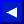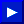### RND

TYPE: Floating-Point Function
FORMAT: RND (<numeric>)

Action: RND creates a floating-point random from 0.0 to 1.0. The computer generates a sequence of random numbers by performing calculations on a starting number, which in computer jargon is called a seed. The RND function is seeded on system power-up. The <numeric> argument is a dummy, except for its sign (positive, zero, or negative).

If the <numeric> argument is positive, the same "pseudorandom" sequence of numbers is returned, starting from a given seed value. Different number sequences will result from different seeds, but any sequence is repeatable by starting from the same seed number. Having a known sequence of "random" numbers is useful in testing programs.

If you choose a <numeric> argument of zero, then RND generates a number directly from a free-running hardware clock (the system "jiffy clock"). Negative arguments cause the RND function to be re-seeded with each function call.

EXAMPLES of RND Function:
```   220 PRINT INT(RND(0)*50)               (Return random integers 0-49)

100 X=INT(RND(1)*6)+INT(RND(1)*6)+2    (Simulates 2 dice)

100 X=INT(RND(1)*1000)+1               (Random integers from 1-1000)

100 X=INT(RND(1)*150)+100              (Random numbers from 100-249)

100 X=RND(1)*(U-L)+L                   (Random numbers between
upper (U) and lower (L) limits)
```This page has been created by Sami Rautiainen. Read the small print. Last updated November 14, 1998.